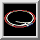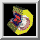#Fundamental Particles and the Standard Model

In recent years, high energy physicists have arrived at a picture of the microscopic physical universe, called "The Standard Model", which unifies the nuclear, electromagnetic, and weak forces and enumerates the fundamental building blocks of the universe:

6 leptons:
• electron, electron neutrino
• muon, muon neutrino
• tau, tau neutrino
6 quarks:
• d (down), u (up)
• s (strange), c (charm)
• b (bottom), t (top)

Ordinary matter is made of protons (each a u-u-d quark triplet), neutrons (each a u-d-d quark triplet), and electrons. Quarks cannot exist singly (or so it appears), so the particles created in accelerator collisions include mesons (combinations of a quark and an anti-quark), baryons (combinations of three quarks), and leptons. All but the proton, electron and neutrinos are unstable and decay to the stable particles. In the Standard Model the forces are communicated between particles by the exchange of quanta which behave like particles:

4 intermediate vector bosons:
• gluon (nuclear force)
• photon (electromagnetic force)
• W and Z bosons (weak force)

In principle, the model can predict the outcome of any experiment (or the probabilities of the various possible outcomes), but in most cases of interest the theoretical calculations are too complicated to be carried out with current techniques. The model also has serious flaws--it does not account for gravity, does not explain or predict the masses of the various particles, and requires a number of parameters (particles masses and the "KM-angles") to be measured and inserted into the theory. To guide us in discovering a more complete theory we need to

• look for flaws in the present model by testing it with experiment
• measure the unknown parameters whose values should be predicted by a better theory
• look for new phenomena not predicted in the Standard Model

CESR normally operates at an energy that is "just right" for producing b quarks through the annhilation of an electron and positron into a b quark and an anti b quark. Since the b quarks are heavy and relatively slow moving, they are more amenable to study than the lighter quarks. Experiments at CESR have provided much of the known information about b quarks, and have provided stringent quantitative tests of the hypothesis of gluon exchange in the Standard Model.dsr@lns598.lns.cornell.edu
\$Id: quark.html,v 1.3 2002/08/29 17:58:06 dsr Exp \$